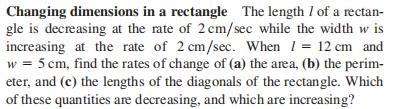### Still have math questions?Changing dimensions in a rectangle The length $$l$$ of a rectan- gle is decreasing at the rate of $$2 cm / sec$$ while the width $$w$$ is increasing at the rate of $$2 cm / sec$$ . When $$l = 12 cm$$ and $$w = 5 cm$$ , find the rates of change of (a) the area, (b) the perim- eter, and (c) the lengths of the diagonals of the rectangle. Which of these quantities are decreasing, and which are increasing?
$$\left. \begin{array} { l } { \text { (a) } 14 cm ^ { 2 } / sec \text { , increasing } \text { (b) } 0 cm / sec \text { , constant } } \\ { \text { (c) } - 14 / 13 cm / sec \text { , decreasing } } \end{array} \right.$$